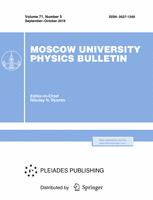Faculty of Physics
M.V.Lomonosov Moscow State University
Regular Article

# Spectral, spatial-energy and polarization characteristics of laser diodes with emissionwavelength of 530 nm

## V.V. Bliznyuk$^1$, N.V. Berezovskaja$^1$, V.A. Parshin$^1$, I.S. Gadaev$^1$, O.I. Koval$^1$, A.G. Rzhanov$^2$, G.A. Solovyev$^1$

### Memoirs of the Faculty of Physics 2015. N 4.

• Article
Annotation

Experimentally investigated the characteristics of laser diodes (LD) operating at the wavelength of 530 nm. It is established that the contrast of the radiation power of the LD is small (less than 0.85) and very sensitive to changes in heterostructures. Contrast and radiation divergence (about one degree) are compared with the spectral characteristics of the LD.

PACS:
42.55.Px Semiconductor lasers; laser diodes
Authors
V.V. Bliznyuk$^1$, N.V. Berezovskaja$^1$, V.A. Parshin$^1$, I.S. Gadaev$^1$, O.I. Koval$^1$, A.G. Rzhanov$^2$, G.A. Solovyev$^1$
$^1$ Department ofPhysics, Institute of Radio Engineering and Electronics, National Research University«MPEI». Moscow 111250, Russia. Krasnokazarmennayast., 17.
$^2$Faculty of Physics, Lomonosov Moscow State University.Moscow 119991, Russia.Leninskie Gory,1, b.2.### Moscow University Physics Bulletin### Science News of the Faculty of Physics, Lomonosov Moscow State University

This new information publication, which is intended to convey to the staff, students and graduate students, faculty colleagues and partners of the main achievements of scientists and scientific information on the events in the life of university physicists.